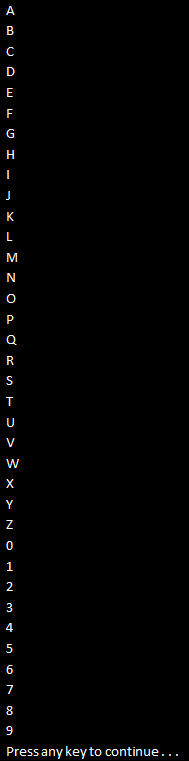HI WELCOME TO SIRING

# Part 8 - SelectMany Operator in LINQ

SelectMany Operator belong to Projection Operators category. It is used to project each element of a sequence to an IEnumerable<T> and flattens the resulting sequences into one sequence.

Let us understand this with an example. Consider the following Student class. Subjects property in this class is a collection of strings.

public class Student
{
public string Name { get; set; }
public string Gender { get; set; }
public List<string> Subjects { get; set; }
public static List<Student> GetAllStudetns()
{
List<Student> listStudents = new List<Student>
{
new Student
{
Name = "Tom",
Gender = "Male",
Subjects = new List<string> { "ASP.NET", "C#" }
},
new Student
{
Name = "Mike",
Gender = "Male",
Subjects = new List<string> { "ADO.NET", "C#", "AJAX" }
},
new Student
{
Name = "Pam",
Gender = "Female",
Subjects = new List<string> { "WCF", "SQL Server", "C#" }
},
new Student
{
Name = "Mary",
Gender = "Female",
Subjects = new List<string> { "WPF", "LINQ", "ASP.NET" }
},
};
return listStudents;
}
}

Example 1: Projects all subject strings of a given a student to an IEnumerable<string>. In this example since we have 4 students, there will be 4 IEnumerable<string> sequences, which are then flattened to form a single sequence i.e a single IEnumerable<string> sequence.

IEnumerable<string> allSubjects = Student.GetAllStudetns().SelectMany(s => s.Subjects);
foreach (string subject in allSubjects)
{
Console.WriteLine(subject);
}

Output:Example 2: Rewrite Example1 using SQL like syntax. When using SQL like syntax style, we don't use SelectMany, instead we will have an additional from clause, which will get it's data from the results of the first from clause.

IEnumerable<string> allSubjects = from student in Student.GetAllStudetns()
from subject in student.Subjects
select subject;
foreach (string subject in allSubjects)
{
Console.WriteLine(subject);
}

Output:
Same output as in Example 1

Example 3: Projects each string to an IEnumerable<char>. In this example since we have 2 strings, there will be 2 IEnumerable<char> sequences, which are then flattened to form a single sequence i.e a single IEnumerable<char> sequence.

string[] stringArray =
{
"ABCDEFGHIJKLMNOPQRSTUVWXYZ",
"0123456789"
};
IEnumerable<char> result = stringArray.SelectMany(s => s);
foreach (char c in result)
{
Console.WriteLine(c);
}

Output:Example 4: Rewrite Example3 using SQL like syntax.
string[] stringArray =
{
"ABCDEFGHIJKLMNOPQRSTUVWXYZ",
"0123456789"
};
IEnumerable<char> result = from s in stringArray
from c in s
select c;
foreach (char c in result)
{
Console.WriteLine(c);
}

Output:
Same output as in Example 3

Example 5: Selects only the distinct subjects
IEnumerable<string> allSubjects = Student.GetAllStudetns()
.SelectMany(s => s.Subjects).Distinct();
foreach (string subject in allSubjects)
{
Console.WriteLine(subject);
}

Output:Example 6: Rewrite Example 5 using SQL like syntax.
IEnumerable<string> allSubjects = (from student in Student.GetAllStudetns()
from subject in student.Subjects
select subject).Distinct();
foreach (string subject in allSubjects)
{
Console.WriteLine(subject);
}

Output:
Same output as in Example 5

Example 7: Selects student name along with all the subjects
var result = Student.GetAllStudetns().SelectMany(s => s.Subjects, (student, subject) =>
new { StudentName = student.Name, Subject = subject });
foreach (var v in result)
{
Console.WriteLine(v.StudentName + " - " + v.Subject);
}

Output:Example 8: Rewrite Example 7 using SQL like syntax.
var result = from student in Student.GetAllStudetns()
from subject in student.Subjects
select new { StudnetName = student.Name, Subject = subject };
foreach (var v in result)
{
Console.WriteLine(v.StudnetName + " - " + v.Subject);
}

Output:
Same output as in Example 7